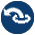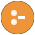#assoc.pl -- Binary associations

Assocs are Key-Value associations implemented as a balanced binary tree (AVL tree).

author
- R.A.O'Keefe, L.Damas, V.S.Costa and Jan Wielemaker
- library(pairs), library(rbtrees)
empty_assoc(?Assoc) is semidet
Is true if Assoc is the empty association list.
assoc_to_list(+Assoc, -Pairs) is det
Translate Assoc to a list Pairs of Key-Value pairs. The keys in Pairs are sorted in ascending order.
assoc_to_keys(+Assoc, -Keys) is det
True if Keys is the list of keys in Assoc. The keys are sorted in ascending order.
assoc_to_values(+Assoc, -Values) is det
True if Values is the list of values in Assoc. Values are ordered in ascending order of the key to which they were associated. Values may contain duplicates.
is_assoc(+Assoc) is semidet
True if Assoc is an association list. This predicate checks that the structure is valid, elements are in order, and tree is balanced to the extent guaranteed by AVL trees. I.e., branches of each subtree differ in depth by at most 1.
gen_assoc(?Key, +Assoc, ?Value) is nondet
True if Key-Value is an association in Assoc. Enumerates keys in ascending order on backtracking.
- get_assoc/3.
get_assoc(+Key, +Assoc, -Value) is semidet
True if Key-Value is an association in Assoc.
Errors
- `type_error(assoc, Assoc)` if Assoc is not an association list.
get_assoc(+Key, +Assoc0, ?Val0, ?Assoc, ?Val) is semidet
True if Key-Val0 is in Assoc0 and Key-Val is in Assoc.
list_to_assoc(+Pairs, -Assoc) is det
Create an association from a list Pairs of Key-Value pairs. List must not contain duplicate keys.
Errors
- `domain_error(unique_key_pairs, List)` if List contains duplicate keys
ord_list_to_assoc(+Pairs, -Assoc) is det
Assoc is created from an ordered list Pairs of Key-Value pairs. The pairs must occur in strictly ascending order of their keys.
Errors
- `domain_error(key_ordered_pairs, List)` if pairs are not ordered.
map_assoc(:Pred, +Assoc) is semidet
True if Pred(Value) is true for all values in Assoc.
map_assoc(:Pred, +Assoc0, ?Assoc) is semidet
Map corresponding values. True if Assoc is Assoc0 with Pred applied to all corresponding pairs of of values.
max_assoc(+Assoc, -Key, -Value) is semidet
True if Key-Value is in Assoc and Key is the largest key.
min_assoc(+Assoc, -Key, -Value) is semidet
True if Key-Value is in assoc and Key is the smallest key.
put_assoc(+Key, +Assoc0, +Value, -Assoc) is det
Assoc is Assoc0, except that Key is associated with Value. This can be used to insert and change associations.
del_min_assoc(+Assoc0, ?Key, ?Val, -Assoc) is semidet
True if Key-Value is in Assoc0 and Key is the smallest key. Assoc is Assoc0 with Key-Value removed. Warning: This will succeed with no bindings for Key or Val if Assoc0 is empty.
del_max_assoc(+Assoc0, ?Key, ?Val, -Assoc) is semidet
True if Key-Value is in Assoc0 and Key is the greatest key. Assoc is Assoc0 with Key-Value removed. Warning: This will succeed with no bindings for Key or Val if Assoc0 is empty.
del_assoc(+Key, +Assoc0, ?Value, -Assoc) is semidet
True if Key-Value is in Assoc0. Assoc is Assoc0 with Key-Value removed.